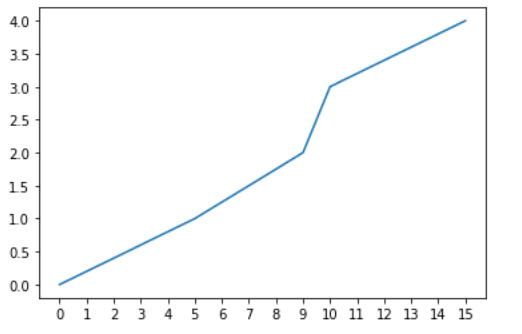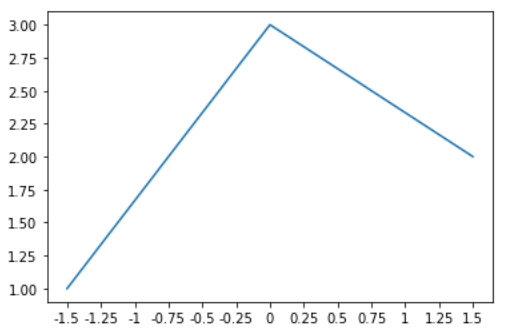# Matplotlib.ticker.MultipleLocator Class in Python

Matplotlib is an amazing visualization library in Python for 2D plots of arrays. Matplotlib is a multi-platform data visualization library built on NumPy arrays and designed to work with the broader SciPy stack.

## matplotlib.ticker.MultipleLocator

The `matplotlib.ticker.MultipleLocator` class is used for setting a tick for every integer multiple of a base within the view interval.

Syntax: class matplotlib.ticker.MultipleLocator(base=1.0)

Methods of the class:

• set_params(self, base): It is used for setting the parameters within the locator.
• tick_values(self, vmin, vmax): For a given vmin and vmax it returns the values of the located ticks.
• view_limits(self, dmin, dmax): It is used for setting the view limits to the nearest multiples of base that contain the data.

Example 1:

 `import` `matplotlib.pyplot as plt ` `import` `matplotlib.ticker as ticker ` ` `  ` `  `x ``=` `[``0``, ``5``, ``9``, ``10``, ``15``] ` `y ``=` `[``0``, ``1``, ``2``, ``3``, ``4``] ` ` `  `tick_spacing ``=` `1` ` `  `fig, ax ``=` `plt.subplots(``1``, ``1``) ` `ax.plot(x, y) ` `ax.xaxis.set_major_locator(ticker.MultipleLocator(tick_spacing)) ` ` `  `plt.show() `

Output:Example 2:

 `import` `matplotlib.pyplot as plt ` `import` `matplotlib.ticker ` ` `  ` `  `plt.plot([``-``1.5``, ``0``, ``1.5``], [``1``, ``3``, ``2``]) ` `ax ``=` `plt.gca() ` ` `  `func ``=` `lambda` `x, pos: ``str``(x).rstrip(``'0'``).rstrip(``'.'``) ` ` `  `ax.xaxis.set_major_locator(matplotlib.ticker.MultipleLocator(``0.25``)) ` `ax.xaxis.set_major_formatter(matplotlib.ticker.FuncFormatter(func)) ` ` `  `plt.show() `

Output:My Personal Notes arrow_drop_upDeveloper with a dream

If you like GeeksforGeeks and would like to contribute, you can also write an article using contribute.geeksforgeeks.org or mail your article to contribute@geeksforgeeks.org. See your article appearing on the GeeksforGeeks main page and help other Geeks.

Please Improve this article if you find anything incorrect by clicking on the "Improve Article" button below.

Article Tags :

Be the First to upvote.

Please write to us at contribute@geeksforgeeks.org to report any issue with the above content.